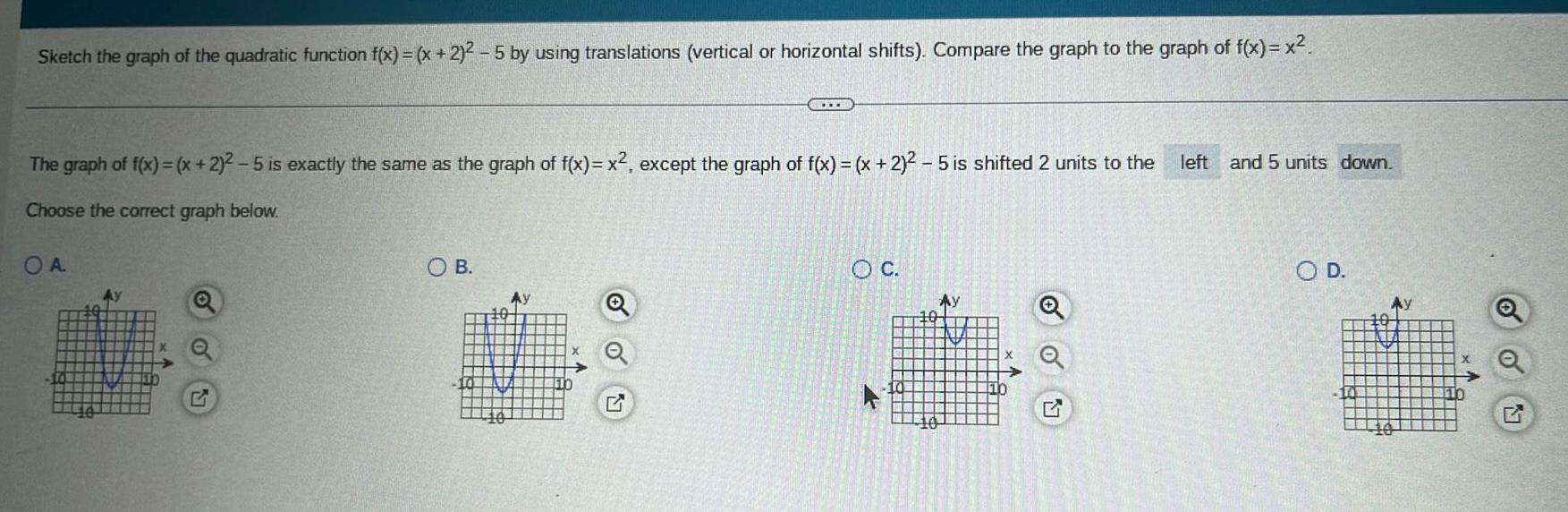Question:

# Sketch the graph of the quadratic function f x x 2 2 5 by

Last updated: 9/14/2023Sketch the graph of the quadratic function f x x 2 2 5 by using translations vertical or horizontal shifts Compare the graph to the graph of f x x The graph of f x x 2 2 5 is exactly the same as the graph of f x x except the graph of f x x 2 5 is shifted 2 units to the left and 5 units down Choose the correct graph below OA Ay SOB 10 Ay www 10 O C P Ay 10 O D 110 10 Ay X Q